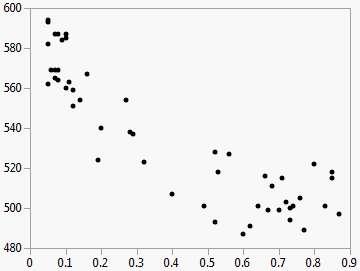Basic Analysis > Bivariate Analysis > Example of Bivariate Analysis
Publication date: 05/24/2021

# Example of Bivariate Analysis

Learn how to construct a scatterplot for two continuous variables. This example uses the SAT.jmp sample data table. SAT test scores for students in the 50 U.S. states, plus the District of Columbia, are divided into two areas: verbal and math. You want to find out how the percentage of students taking the SAT tests is related to verbal test scores for 2004.

1. Select Help > Sample Data Library and open SAT.jmp.

2. Select Analyze > Fit Y by X.

3. Select 2004 Verbal and click Y, Response.

4. Select % Taking (2004) and click X, Factor.

5. Click OK.

Figure 5.2 Example of SAT Scores by Percent TakingYou can see that the verbal scores were higher when a smaller percentage of the population took the test.